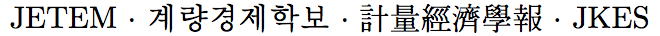Journal of Economic Theory and Econometrics: Journal of the Korean Econometric Society
Home

Archive

Search

Journal of Economic Theory and EconometricsJournal of the Korean Econometric Society

• #### Dong Chul Won   (Ajou University, School of Business)Abstract

The paper is a sequel to Won (2016) which attempts to characterize full-rank GEI equilibrium as pre-GEI equilibrium. The pre-GEI equilibrium approach for computing full-rank GEI equilibrium is useful because pre-GEI equilibrium always exist under standard conditions. The goal of the current paper is to develop algorithms which can be implemented to compute pre-GEI equilibrium. The algorithms are built on a prototype system of equations which consist of the first-order conditions for utility maximization and market clearing conditions. The prototype system can be directly encoded into an algorithm or can be transformed into implementable forms for algorithms such as homotopy path-following algorithms. Two examples are presented where the algorithms are implemented to compute pre-GEI equilibrium and their performance are comparatively discussed.

Keywords
incomplete markets, pre-GEI equilibrium, real assets, homotopy path-following algorithms, quasi-solution.

JEL classification codes
D52, D53, C62, C63, C02